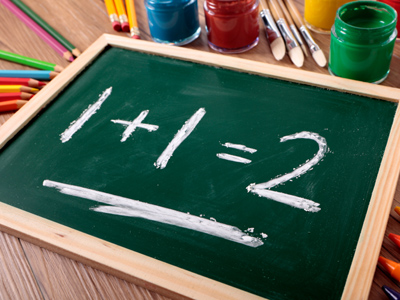One plus one equals two.

# Year 1 Calculation - Symbols

This quiz addresses the requirements of the National Curriculum KS1 Maths and Numeracy for children aged 5 and 6 in year 1. Specifically this quiz is aimed at the section dealing with reading, writing and interpreting mathematical statements involving addition (+), subtraction (–) and equals (=) signs.

Recognising, selecting and using the right symbol in a number sentence is vital. A good understanding of what the addition, subtraction and equals signs look like, what they mean in maths, and what they do to numbers is a really important area of development. Understanding the language associated with the addition, subtraction and equals signs will help children calculate accurately.

Question 1
Which symbol is missing from this calculation:
3 ? 6 = 9
+
-
=
x
Question 2
Which symbol is missing from this calculation:
4 ? 1 = 3
÷
=
+
-
Subtracting 1 from 4 gives an answer of 3
Question 3
What is wrong with this number sentence:
7 + 3 = 4
The addition sign should be a subtraction sign
The addition sign is in the wrong place
The equal sign is in the wrong place
The numbers are too small
If you change - for +, the answer becomes correct
Question 4
What does this symbol mean: +
Subtracting or subtraction
Is the same as
It is used when numbers are added together, for example 4 + 5 = 9
Question 5
Which symbol could be used instead of the words in brackets?
5 (plus) 5 = 10
-
=
+
÷
Plus is another word for add
Question 6
Which symbol could be used instead of the words in brackets?
5 + 4 (is equal to) 9
+
=
-
x
This symbol means 'is equal to' or 'is the same as'
Question 7
What does this symbol mean: -
Multiplication
Subtracting or subtraction
Division
This symbol is used in subtraction calculations, such as 5 - 3 = 2
Question 8
What does this symbol mean: =
Is equal to or the same as
Dividing
Multiplying
Many people think this symbol means 'is the answer' but it really means 'is equal to'
Question 9
Which symbol is missing from this calculation:
6 - 2 ? 4
x
+
=
-
6 - 2 is equal to, or the same as 4
Question 10
Which symbol could be used instead of the words in brackets?
5 (minus) 5 = 0
x
=
+
-
Minus is another way of saying subtract or take away